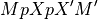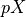Note

This page is a reference documentation. It only explains the class signature, and not how to use it. Please refer to the user guide for the big picture.

3.5.1. nistats.model.LikelihoodModelResults¶

class nistats.model.LikelihoodModelResults(theta, Y, model, cov=None, dispersion=1.0, nuisance=None, rank=None)

Class to contain results from likelihood models

__init__(self, theta, Y, model, cov=None, dispersion=1.0, nuisance=None, rank=None)

Set up results structure

Parameters
thetandarray

parameter estimates from estimated model

Yndarray

data

modelLikelihoodModel instance

model used to generate fit

covNone or ndarray, optional

covariance of thetas

dispersionscalar, optional

multiplicative factor in front of cov

nuisanceNone of ndarray

parameter estimates needed to compute logL

rankNone or scalar

rank of the model. If rank is not None, it is used for df_model instead of the usual counting of parameters.

Notes

The covariance of thetas is given by:

dispersion * cov

For (some subset of models) dispersion will typically be the mean square error from the estimated model (sigma^2)

Fcontrast(self, matrix, dispersion=None, invcov=None)

Compute an Fcontrast for a contrast matrix matrix.

Here, matrix M is assumed to be non-singular. More preciselyis assumed invertible. Here,is the generalized inverse of the design matrix of the model. There can be problems in non-OLS models where the rank of the covariance of the noise is not full.

See the contrast module to see how to specify contrasts. In particular, the matrices from these contrasts will always be non-singular in the sense above.

Parameters
matrix1D array-like

contrast matrix

dispersionNone or float, optional

If None, use self.dispersion

invcovNone or array, optional

Known inverse of variance covariance matrix. If None, calculate this matrix.

Returns
f_resFContrastResults instance

with attributes F, df_den, df_num

Notes

For F contrasts, we now specify an effect and covariance

Tcontrast(self, matrix, store=('t', 'effect', 'sd'), dispersion=None)

Compute a Tcontrast for a row vector matrix

To get the t-statistic for a single column, use the ‘t’ method.

Parameters
matrix1D array-like

contrast matrix

storesequence, optional

components of t to store in results output object. Defaults to all components (‘t’, ‘effect’, ‘sd’).

dispersionNone or float, optional
Returns
resTContrastResults object
conf_int(self, alpha=0.05, cols=None, dispersion=None)

The confidence interval of the specified theta estimates.

Parameters
alphafloat, optional

The alpha level for the confidence interval. ie., alpha = .05 returns a 95% confidence interval.

colstuple, optional

cols specifies which confidence intervals to return

dispersionNone or scalar

scale factor for the variance / covariance (see class docstring and vcov method docstring)

Returns
cisndarray

cis is shape (len(cols), 2) where each row contains [lower, upper] for the given entry in cols

Notes

Confidence intervals are two-tailed.

tailsstring, optional

Possible values: ‘two’ | ‘upper’ | ‘lower’

Examples

>>> from numpy.random import standard_normal as stan
>>> from nistats.regression import OLSModel
>>> x = np.hstack((stan((30,1)),stan((30,1)),stan((30,1))))
>>> beta=np.array([3.25, 1.5, 7.0])
>>> y = np.dot(x,beta) + stan((30))
>>> model = OLSModel(x).fit(y)
>>> confidence_intervals = model.conf_int(cols=(1,2))
logL(self)

The maximized log-likelihood

t(self, column=None)

Return the (Wald) t-statistic for a given parameter estimate.

Use Tcontrast for more complicated (Wald) t-statistics.

vcov(self, matrix=None, column=None, dispersion=None, other=None)

Variance/covariance matrix of linear contrast

Parameters
matrix: (dim, self.theta.shape) array, optional

numerical contrast specification, where dim refers to the ‘dimension’ of the contrast i.e. 1 for t contrasts, 1 or more for F contrasts.

column: int, optional

alternative way of specifying contrasts (column index)

dispersion: float or (n_voxels,) array, optional

value(s) for the dispersion parameters

other: (dim, self.theta.shape) array, optional

alternative contrast specification (?)

Returns
cov: (dim, dim) or (n_voxels, dim, dim) array

the estimated covariance matrix/matrices

Returns the variance/covariance matrix of a linear contrast of the
estimates of theta, multiplied by dispersion which will often be an
estimate of dispersion, like, sigma^2.
The covariance of interest is either specified as a (set of) column(s)
or a matrix.